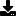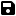Don't have an account yet? Register

# Validate phone number, email address and south african ID number

by Chris Morton (5 Submissions)
Category: String Manipulation
Compatability: VB.NET
Difficulty: Unknown Difficulty
Originally Published: Sun 16th October 2005
Date Added: Mon 8th February 2021
Rating:(1 Votes)

Validate phone number, email address and south african ID number

Rate Validate phone number, email address and south african ID number

`    Public Function ValidateEmail(ByVal Email As String) As Boolean        Dim i As Integer        Dim atplace As Integer        Dim dotplace As Integer        Dim atcount As Integer        Dim atcountboo As Boolean        Dim dotcount As Integer        For i = 1 To Len(Email)            If Mid(Email, i, 1) = "@" Then 'Checks where the location of the @ symbol is and if there is more than 1                atcount = atcount + 1                atplace = i                If atcount > 1 Then                    Return False                End If            End If            If Mid(Email, i, 1) = "." Then                dotcount = dotcount + 1                dotplace = i            End If            If InStr("[email protected]_", LCase(Mid(Email, i, 1))) > 0 Then 'checks for illegal charaters            Else                Return False            End If        Next        If dotplace < atplace Or Len(Email) = dotplace Then 'checks to see whether the is a . after the @ and if something follows after the .            Return False        End If        If atcount <> 1 Then 'checks if there are invalid or no @ symbols            atcountboo = False        Else            atcountboo = True        End If        If Mid(Email, 1, 1) = "@" Or Mid(Email, Len(Email), 1) = "@" Then 'checks if the first or last charater is @            Return False        End If        If Mid(Email, atplace + 1, 1) = "." Then 'checks if the character after @ is a .            Return False        End If        If dotcount >= 1 And atcountboo = True Then 'checks if there is at least 1 . and an @ exists in the right place            Return True        Else            Return False        End If    End Function    Public Function ValidateIDNumber(ByVal IDNumber As String, ByVal DateofBirth As Date) As Boolean        Dim First6Numbers() As String        Dim i As Integer        Dim numbers(2) As Integer        Dim DOB As Integer        If IsNumeric(IDNumber) = True And Len(IDNumber) = 13 Then        Else            Return False        End If        First6Numbers = Split(DateofBirth.ToShortDateString, "/")        For i = 0 To First6Numbers.Length - 1            numbers(i) = First6Numbers(i)        Next        DOB = Mid(Format(numbers(0), "0000") & Format(numbers(1), "00") & Format(numbers(2), "00"), 3, 6)        If Mid(IDNumber, 1, 6) = DOB Then            Return True        Else            Return False        End If    End Function    Public Function ValidatePhoneNumber(ByVal PhoneNumber As String) As Boolean        Dim i As Integer        Dim pluscount As Integer        For i = 1 To Len(PhoneNumber)            If InStr("1234567890- +()", LCase(Mid(PhoneNumber, i, 1))) > 0 Then 'checks for illegal charaters            Else                Return False            End If        Next        For i = 1 To Len(PhoneNumber)            If Mid(PhoneNumber, i, 1) = "+" Then 'Checks if there is more than 1 "+"                pluscount += 1                If pluscount > 1 Then                    Return False                End If            End If        Next        Return True    End FunctionEnd Module`Download this snippetAdd to My Saved Code

# Validate phone number, email address and south african ID number Comments

No comments have been posted about Validate phone number, email address and south african ID number. Why not be the first to post a comment about Validate phone number, email address and south african ID number.

# Post your comment

Subject:
Message:
0/1000 characters